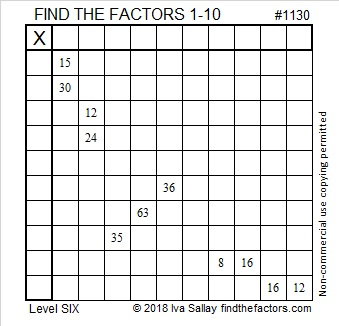# 1130 and Level 6

Today’s puzzle has four sets of clues with two or more common factors. Which common factor should you choose in each case? Guessing and checking will only frustrate you. Study the puzzle instead. The clues work together to give ONE logical solution.Print the puzzles or type the solution in this excel file: 10-factors-1121-1133

Now here is some information about the number 1130:

• 1130 is a composite number.
• Prime factorization: 1130 = 2 × 5 × 113
• The exponents in the prime factorization are 1, 1, and 1. Adding one to each and multiplying we get (1 + 1)(1 + 1)(1 + 1) = 2 × 2 × 2 = 8. Therefore 1130 has exactly 8 factors.
• Factors of 1130: 1, 2, 5, 10, 113, 226, 565, 1130
• Factor pairs: 1130 = 1 × 1130, 2 × 565, 5 × 226, or 10 × 113
• 1130 has no square factors that allow its square root to be simplified. √1130 ≈ 33.615471130 is the hypotenuse of FOUR Pythagorean triples:
150-1120-1130 which is 10 times (15-112-113)
552-986-1130 which is 2 times (276-493-565)
678-904-1130 which is (3-4-5) times 226
792-806-1130 which is 2 times (396-403-565)

1130 is also a leg in some Pythagorean triples including
1130-12744-12794 calculated from 2(113)(5), 113² – 5², 113² + 5²

1130 is palindrome 505 in BASE 15 because 5(15²) + 5(1) = 5(226) = 1130

This site uses Akismet to reduce spam. Learn how your comment data is processed.